The CircleCustom SearchTHE CIRCLE A circle is the locus of all points, in a plane that is a fixed distance from a fixed point, called the center. The fixed distance spoken of here is the radius of the circle. The equation of a circle with its center at the origin (fig. 2-5) is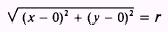where (x,y) is a point on the circle and r is the radius (r replaces d in the standard distance formula). Then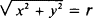or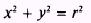Figure 2-5.-Circle with center at the origin. If the center of a circle, figure 2-6, is at some point where x = h, y = k, then the distance of the point (x,y) from the center will be constant and equal to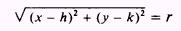or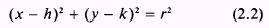Figure 2-6.-Circle with center at (h,k). Equations (2.1) and (2.2) are the standard forms for the equation of a circle. Equation (2.1) is merely a special case of equation (2.2) in which h and k are equal to zero. The equation of a circle may also be expressed in the general form: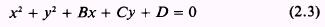where B, C, and D are constants. Theorem: An equation of the second degree in which the coefficients of the x2 and y2 terms are equal and the xy term does not exist, represents a circle. Whenever we find an equation in the form of equation (2.3), we should convert it to the form of equation (2.2) so that we have the coordinates of the center of the circle and the radius as part of the equation. This may be done as shown in the following example problems: EXAMPLE: Find the coordinates of the center and the radius of the circle described by the following equation: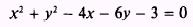SOLUTION: First rearrange the termsand complete the square in both x and y. Completing the square is discussed in the chapter on quadratic solutions in Mathematics, Volume 1. The procedure consists basically of adding certain quantities to both sides of a second-degree equation to form the sum of two perfect squares. When both the first- and second�degree members are known, the square of one-half the coefficient of the first-degree term is added to both sides of the equation. This will allow the quadratic equation to be factored into the sum of two perfect squares. To complete the square in x in the given equationadd the square of one-half the coefficient of x to both sides of the equation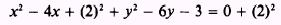then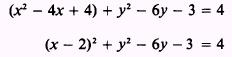completes the square in x. If we do the same for y,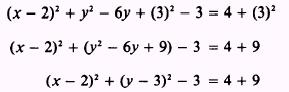completes the square in y. Transpose all constant terms to the right-hand side and simplify: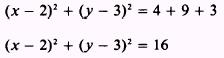The equation is now in the standard form of equation (2.2). This equation represents a circle with the center at (2,3) and with a radius equal to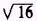or 4. EXAMPLE: Find the coordinates of the center and the radius of the circle given by the equation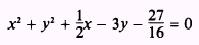SOLUTION: Rearrange and complete the square in both x and y: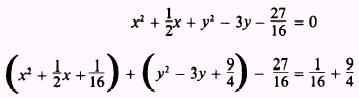Transposing all constant terms to the right-hand side and adding, results in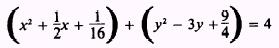Reducing to the equation in standard form results in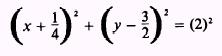Integrated Publishing, Inc. - A (SDVOSB) Service Disabled Veteran Owned Small Business